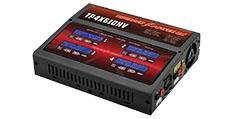# LiPo Charging - Understanding Ohms Law and the limitationsLiPo Charging - Understanding Ohm's Law and the limitations from Cyclone FPV

I am posting this quick reference but will be adding a video to help make it easier. Please make sure to check for the video this coming week (01/27/20)

When trying to figure out your max charging rate of a LiPo or perhaps the configuration you need in order to achieve your desired max charging rate, you must refer to Ohm's Law:

1. V = i * R (voltage = current (amps) * resistance (ohms))
2. i = P / V (current (amps) = power (watts) / voltage)
3. P = i * V (power (watts) = current (amps) * voltage)

So here are some examples:

• Example #1 6S LiPo 6000mAh on 100W Charger (find max charge rate)
• (use equation #2 from above to solve this)
1. Take cell count and multiply by 4.2v (6 x 4.2 = 25.2V)
2. Now plug in the numbers
• i = 100W / 25.2 = 3.968 (or 4A)
• This means that if you are charging a 6S LiPo on a 100W charger, the max charge rate for this battery setup is 4A.

This was a quick explanation in order to assist a customer with their question but I will be adding much more details to this shortly. If you have any questions about this, please make sure to email me at tarek@cyclonefpv.com.